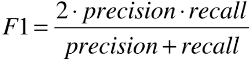# 机器学习：评价分类结果（F1 Score）

2018/07/31 14:29

### 一、基础

• 疑问1：具体使用算法时，怎么通过精准率和召回率判断算法优劣？
• 根据具体使用场景而定：
1. 例1：股票预测，未来该股票是升还是降？业务要求更精准的找到能够上升的股票；此情况下，模型精准率越高越优。
2. 例2：病人诊断，就诊人员是否患病？业务要求更全面的找出所有患病的病人，而且尽量不漏掉一个患者；甚至说即使将正常人员判断为病人也没关系，只要不将病人判断成健康人员就好。此情况，模型召回率越高越优。

• 疑问2：:有些情况下，即需要考虑精准率又需要考虑召回率，二者所占权重一样，怎么中欧那个判断？
• 方法：采用新的评价标准，F1 Score；

### 二、F1 Score

• F1 Score：兼顾降准了和召回率，当急需要考虑精准率又需要考虑召回率，可查看模型的 F1 Score，根据 F1 Score 的大小判断模型的优劣；
• F1 = 2 * Precision * recall / (precision + recall)，是二者的调和平均值；
•1. F1 是 precision 和 recall 的调和平均值
2. 调和平均值：如果 1/a = (1/b + 1/c) / 2，则称 a 是 b 和 c 的调和平均值；
3. 调和平均值特点：|b - c| 越大，a 越小；当 b - c = 0 时，a = b = c，a 达到最大值；
4. 具体到精准率和召回率，只有当二者大小均衡时，F1 指标才高，

### 三、F1 Score 的使用

• F1 Score 指标在 scikit-learn 中封装在了 sklearn.metrics 模块下的 f1_score() 方法中
``````from sklearn.metrics import f1_score

f1_score(y_test, y_log_predict)
# 0.8674698795180723``````

• ``````import numpy as np
from sklearn import datasets

X = digits.data
y = digits.target.copy()

y[digits.target==9] = 1
y[digits.target!=9] = 0

from sklearn.model_selection import train_test_split
X_train, X_test, y_train, y_test = train_test_split(X, y, random_state=666)

from sklearn.linear_model import LogisticRegression
log_reg = LogisticRegression()
log_reg.fit(X_train, y_train)
y_log_predict = log_reg.predict(X_test)

from sklearn.metrics import precision_score
precision_score(y_test, y_log_predict)
# 精准率：0.9473684210526315

from sklearn.metrics import recall_score
recall_score(y_test, y_log_predict)
# 召回率：0.8

from sklearn.metrics import f1_score
f1_score(y_test, y_log_predict)
# F1 Score 指标：0.8674698795180723``````

• 使用scikit-learn 中 sklearn.metrics 模块下的 confusion_matrix()precision_score()recall_score()f1_score() 方法时，所需要的参数都是 y_testy_predict

0
0 收藏

### 作者的其它热门文章0 评论
0 收藏
0